# Trigonometry Formula

Here we will learn about trigonometry formulas, including the three trigonometric functions; the sine function, \text{Sin}, the cosine function, \text{Cos}, and the tangent function, \text{Tan}.

We will also look at the inverse trigonometric functions, the sine rule, cosine rule and the trigonometric formula for the area of a triangle.

There are also trigonometry formulas worksheets based on Edexcel, AQA and OCR exam questions, along with further guidance on where to go next if you’re still stuck.

## What is a trigonometry formula?

A trigonometry formula is a formula that is used to represent relationships between the parts of a triangle including the side lengths, angles and the area.

To solve problems using trigonometric formulas, we need to be able to use the information provided to deduce which trigonometric formula we need to use.

Right-Angled Triangles

\sin{\theta}=\frac{O}{H}

\cos{\theta}=\frac{A}{H}

\tan{\theta}=\frac{O}{A}

Non-Right Angled Triangles

Sine Rule

\frac{a}{\sin{A}} = \frac{b}{\sin{B}} = \frac{c}{\sin{C}}

Cosine Rule

a^2=b^2+c^2-2bc \cos(A)
Area of a Triangle

\text{Area }=\frac{1}{2}ab \sin{(C)}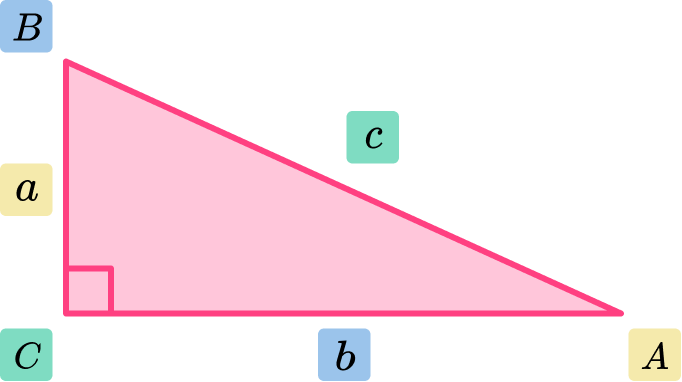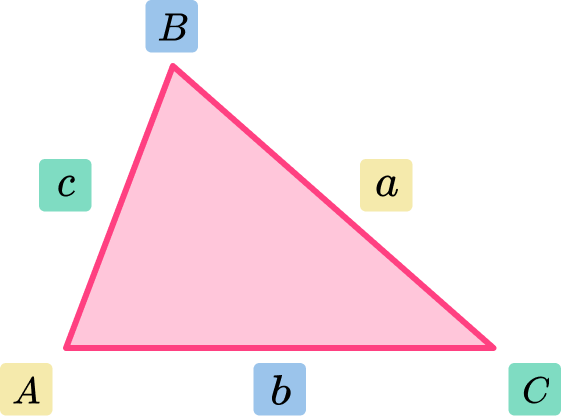### What is a trigonometry formula?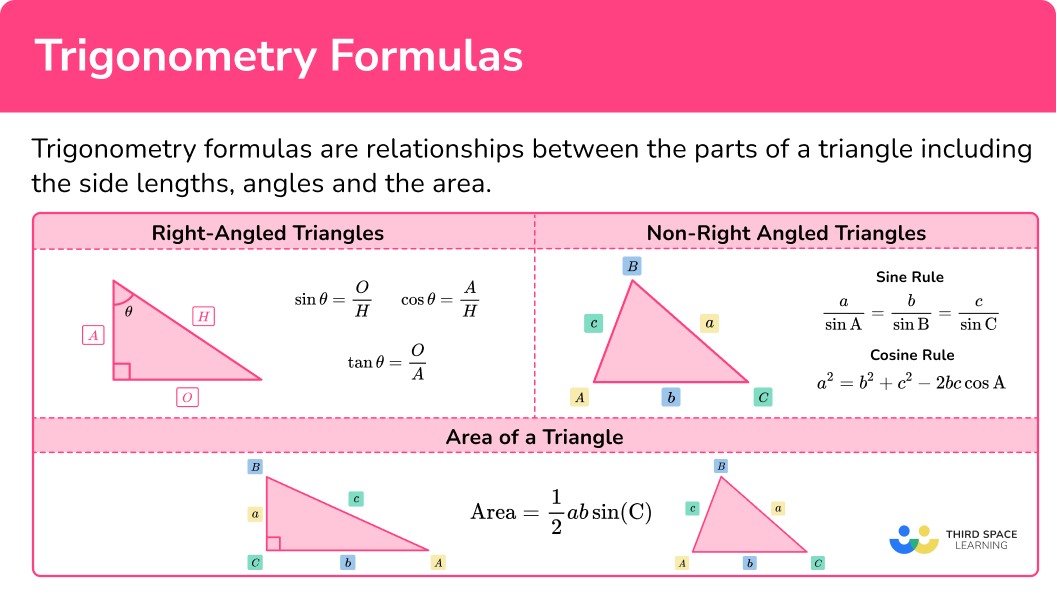### Trigonometry formulas for right-angled triangles

Basic trigonometry focuses on the right-angled triangle.

The three trigonometric functions we use are sine \text{(sin)}, cosine \text{(cos)} and tangent \text{(tan)}. These three functions relate an angle to two sides of a right-angled triangle, giving us the three trigonometric ratios.

Trigonometric FunctionSine

f(x)=\sin(x)
Cosine

f(x)=\cos(x)
Tangent

f(x)=\tan(x)
Trigonometric Ratio \sin{\theta}=\frac{O}{H} \cos{\theta}=\frac{A}{H} \tan{\theta}=\frac{O}{A}

The letter \text{x} represents a value, hence, \text{f(x)} is a function of the value \text{x}.

The symbol \theta represents the angle.

The letter \text{O} represents the opposite side to the angle \theta .

The letter \text{A} represents the adjacent side to the angle \theta .

The letter \text{H} represents the hypotenuse of the right-angled triangle (which is always the side of the triangle facing the right-angle). Also known as the longest side of the triangle.

Example 1

Here,

• the angle \theta=30^{\circ}
• the side opposite the angle O=4 \ cm,
• the unknown length of the hypotenuse H=x \ cm.

We know \theta and O, and we want to find H.

Using SOHCAHTOA, SOH is the trigonometric ratio, so we need to use

\sin(\theta)=\frac{O}{H}.

Example 2

Here,

• the angle \theta=28^{\circ},
• the hypotenuse H=5 \ cm,
• the unknown length of the adjacent side A=x \ cm.

We know \theta and H, and we want to find A.

Using SOHCAHTOA, CAH is the trig ratio, so we need to use

\cos(\theta)=\frac{A}{H}.

Example 3

Here,

• the angle \theta=63^{\circ},
• the adjacent side A=7 \ cm,
• the unknown length of the opposite side O=x \ cm.

We know \theta and A, and we want to find O.

Using SOHCAHTOA, TOA is the trig ratio, so we need to use

\tan(\theta)=\frac{O}{A}.

The solutions are,

Example 1: H=8 \ cm

Example 2: A=4.14 \ cm \ (2dp)

Example 3: O=13.74 \ cm \ (2dp)

Step-by-step guide: SOHCAHTOA

Step-by-step guide: Trigonometric Functions

### Inverse trigonometric functions

When we know two of the three sides of a right angled triangle and we want to find a missing angle, we need to use inverse trigonometric functions.

The three inverse trigonometric functions are

Inverse trigonometric functionInverse Sine

f^{-1}(x)=\sin^{-1}(x)
Inverse Cosine

f^{-1}(x)=\cos^{-1}(x)
Inverse Tangent

f^{-1}(x)=\tan^{-1}(x)
Inverse trig of a ratio \theta=\sin^{-1}(\frac{O}{H}) \theta=\cos^{-1}\left(\frac{A}{H}\right) \theta=\tan^{-1}\left(\frac{O}{A}\right)

Example 1

Here,

• the side opposite the angle O=5 \ cm,
• the length of the hypotenuse H=10 \ cm,
• the unknown angle is \theta.

We know O and H, and we want to find \theta .

Using SOHCAHTOA, SOH is the trig ratio, so as we need to find the value of \theta, we must use the inverse trig formula \theta=\sin^{-1}\left(\frac{O}{H}\right).

Example 2

Here,

• the side adjacent to the angle A=4 \ cm,
• the length of the hypotenuse H=5 \ cm,
• the unknown angle is \theta.

We know A and H, and we want to find \theta .

Using SOHCAHTOA, CAH is the trig ratio, so as we need to find the value of \theta , we must use the inverse trig formula \theta=\cos^{-1}\left(\frac{A}{H}\right).

Example 3

Here,

• the side opposite the angle O=12 \ cm,
• the side adjacent to the angle A=8 \ cm,
• the unknown angle is \theta .

We know O and A, and we want to find \theta .

Using SOHCAHTOA, TOA is the trig ratio, so as we need to find the value of \theta, we must use the inverse trig formula \theta=\tan^{-1}\left(\frac{O}{A}\right).

The solutions are,

Example 1: \theta=30^{\circ}

Example 2: \theta=36.87^{\circ}\text{ (2dp)}

Example 3: \theta=56.31^{\circ} \text{ (2dp)}

Step-by-step guide: Trigonometric functions

### Trigonometric formulas in non-right angled triangles

We can use trigonometry in all types of triangles including scalene, equilateral and isosceles.

Sine rule

The sine rule (sometimes called the law of sines) is the relationship between the sine of an angle, and the side opposite that angle.

More specifically the ratio of the sine of an angle and the opposing side to that angle is the same for all three angles/sides. As a formula, this is written as

\frac{a}{\sin{A}}=\frac{b}{\sin{B}}=\frac{c}{\sin{C}}.

Where a, \ b, and c are the three sides of a triangle, and A, \ B, and C are the angles that face their opposing side (see the diagram below).

Notice that the upper case letters denote the angles, and the lower case letters denote the side lengths where a is opposite A, \ b is opposite B, and c is opposite C.

The equation above shows how all three side lengths, divided by the sine of the angle facing that side, are the same value. We only need to work with a pair of letters, giving us the working formula for the law of sines.

\frac{a}{\sin{A}}=\frac{b}{\sin{B}}

We can use the sine rule to find

• a missing side using this arrangement of the formula.

\frac{a}{\sin{A}}=\frac{b}{\sin{B}}

By rearranging the formula to make the unknown side a the subject, we have

a=\frac{b\sin{A}}{\sin{B}}.

• a missing angle using  this arrangement of the formula.

\frac{\sin{A}}{a}=\frac{\sin{B}}{b}

By rearranging the formula to make the unknown angle \text{A} the subject, we have

A=\sin^{-1}\left(\frac{a\sin{B}}{b}\right).

Step-by-step guide: Sine Rule

Step-by-step guide: Changing the subject

For example,

Below is a non-right angled triangle. We can use the law of sines to find the length \text{AB}.

\begin{aligned} & \frac{a}{\sin A}=\frac{b}{\sin B} \\\\ & \frac{A B}{\sin (28)}=\frac{10.2}{\sin (134)} \\\\ & A B=\sin (28) \times \frac{10.2}{\sin (134)} \\\\ & A B=6.656951191 \mathrm{~cm} \end{aligned}

### Cosine rule

The cosine rule (sometimes called the law of cosines ) is the relationship between the cosine of an angle, and all three sides of the triangle.

As a formula, this is written as

a^{2}=b^{2}+c^{2}-2bc\cos(A).

Where a, \ b, and c are the three sides of a triangle, and A is the angle between the two sides of b and c (see the diagram below).

Notice that the upper case A is an angle, and the lower case letters are the side lengths where a is opposite A, and b and c are the two sides that surround the angle A.

We need to use the cosine rule when we don’t know one of the four values of a, \ b, \ c, or A.

We can use the cosine rule to find

• a missing side by using this arrangement of the formula,

a^{2}=b^{2}+c^{2}-2bc\cos(A).

• a missing angle by using this arrangement of the formula,

A=\cos^{-1}(\frac{b^{2}+c^{2}-a^{2}}{2bc}).

Step-by-step guide: Cosine Rule

Step-by-step guide: Changing the subject

For example,

Below is a non-right angled triangle. We can use the law of cosine to find the length \text{BC}.

\begin{aligned} & a^2=b^2+c^2-2 b c \cos (A) \\\\ & B C^2=4.8^2+6.4^2-2 \times 4.8 \times 6.4 \times \cos (123) \\\\ & B C^2=97.46262231 \\\\ & B C=\sqrt{97.46262231} \\\\ & B C=9.872315955 \mathrm{~cm} \end{aligned}

### Area of a triangle

We can calculate the area of any triangle if we need to know two sides and the included angle between those two sides. The formula is

Area=\frac{1}{2}ab\sin(C).

Where C is the angle between the two sides of a and b (see the diagram below).

We can also find a missing angle knowing the area of the triangle and by rearranging the formula to find the angle \text{C}.

C=\sin^{-1}(\frac{2Area}{ab})

We can find a missing side length knowing the area of the triangle and rearranging the formula to find a missing side length a.

a=\frac{2Area}{b\sin(C)}

Step-by-step guide: Area of a triangle trig

For example,

We can find the area of the triangle below using the trigonometric formula for the area of any triangle.

\begin{aligned} & Area=\frac{1}{2} a b \sin (C) \\\\ & Area=\frac{1}{2} \times 5 \times 7 \times \sin (40) \\\\ & Area=11.24878317 \mathrm{~m}^2 \end{aligned}

### Choosing the correct trigonometric formula

In order to determine which trigonometric formula you need to use, the flow chart below can help you decide.

Remembering that, to find the area of any triangle, we can use the sine rule for area.

## How to solve problems using trigonometry formulas

In order to solve problems using trigonometry formulas:

1. Determine which trigonometry formula is needed to answer the question.
2. Label each side and angle appropriately.
3. Substitute known values into the formula.
4. Solve for the unknown value.

### Explain how to solve problems using trigonometry formulas## Trigonometry formulas examples

### Example 1: SOHCAHTOA (missing side)

Calculate the length of the side labelled x. Write your answer correctly to 2 decimal places.

1. Determine which trigonometry formula is needed to answer the question.

Using the flowchart,

This means that we need to use either of the three trig ratios of sine, cosine or tangent. We can work out which one in the next step.

2Label each side and angle appropriately.

From the angle of 20^{\circ},

• the side opposite the angle O=10 \ cm,
• the hypotenuse H=x \ cm,
• the adjacent side has no value and so is not used.

As we know the opposite side and we want to find the hypotenuse, the trigonometric ratio that we have to use is \sin(\theta)=\frac{O}{H}.

Here, we used the abbreviation \text{SOHCAHTOA}, where \text{SOH} is the sine ratio \text{S}, of the opposite \text{O}, and the hypotenuse \text{H}.

3Substitute known values into the formula.

As O=10, \ H=x, and \theta=20, substituting these values into the formula

\sin(\theta)=\frac{O}{H}, we have

\sin(20)=\frac{10}{x}.

4Solve for the unknown value.

Multiplying both sides by x gives us

x\times\sin(20)=10.

Dividing both sides by \sin(20) gives us

x=\frac{10}{\sin(20)}=29.238044…

This means that the length x=29.24 \ cm \ (2dp).

### Example 2: SOHCAHTOA (missing angle)

Determine the size of the missing angle, marked \theta in the triangle below. Write your answer correctly to 2 decimal places.

Determine which trigonometry formula is needed to answer the question.

Label each side and angle appropriately.

Substitute known values into the formula.

Solve for the unknown value.

### Example 3: SOHCAHTOA

Determine the missing length x of the right-angled triangle below. Write your answer to 2 decimal places.

Determine which trigonometry formula is needed to answer the question.

Label each side and angle appropriately.

Substitute known values into the formula.

Solve for the unknown value.

### Example 4: cosine rule

Determine the length of the side labelled x for the triangle below. Write your answer correctly to 2 decimal places.

Determine which trigonometry formula is needed to answer the question.

Label each side and angle appropriately.

Substitute known values into the formula.

Solve for the unknown value.

### Example 5: sine rule

Determine the size of the missing angle \theta. Write your answer to 2 decimal places.

Determine which trigonometry formula is needed to answer the question.

Label each side and angle appropriately.

Substitute known values into the formula.

Solve for the unknown value.

### Example 6: area using trigonometry formulae

Calculate the area of the triangle below. Write your answer correctly to 2 decimal places.

Determine which trigonometry formula is needed to answer the question.

Label each side and angle appropriately.

Substitute known values into the formula.

Solve for the unknown value.

### Common misconceptions

• Labelling a triangle incorrectly

For example, this triangle has been incorrectly labelled with the side c next to the angle rather than opposite it.

• Using the incorrect trigonometric function

If the triangle is incorrectly labelled, it can lead to the use of the incorrect standard or inverse trigonometric functions and formulae.

• Rounding the decimal too early

This can lose accuracy marks. Always use as many decimal places as possible throughout the calculation, then round the final solution.

• Using the sine rule instead of the cosine rule

In order to use the sine rule, we need to have pairs of opposite angles and sides.

• Not using the included angle

For the cosine rule and the area of a triangle using A=\frac{1}{2}ab\sin(C), the angle is included between the two sides. Using any other angle will result in an incorrect solution.

• Using \bf{A=b\times{h}\div{2}}

If the vertical height of a triangle is not available then we cannot calculate the area by halving the base times the height.

• Using the inverse trig function instead, inducing a mathematical error

If the inverse trig function is used instead of the standard trig function, the calculator may return a maths error as the solution does not exist.

• Trigonometry can only be used in right-angled triangles

The use of trigonometry does not have to be limited to a right-angled triangle where all angles are acute angles. We can also use the law of cosines, the law of sines, and the area of a triangle using the sine rule on triangles that are not right-angled.

### Practice trigonometry formulas questions

1. Determine the size of the missing side marked x in the triangle below. Write your answer to 2 decimal places.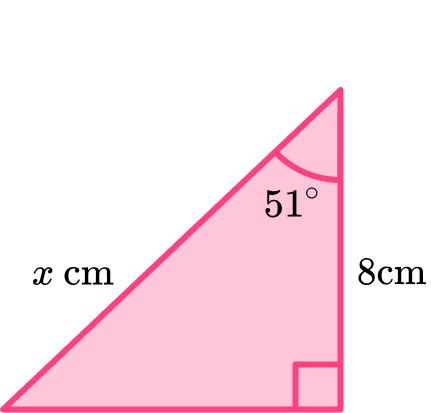x=5.03 \ cmx=6.22 \ cmx=10.29 \ cmx=12.71 \ cmAfter labelling the triangle with O, \ A and H,

• A=8 \ cm
• H=x \ cm
• \theta=51^{\circ}

SOH CAH TOA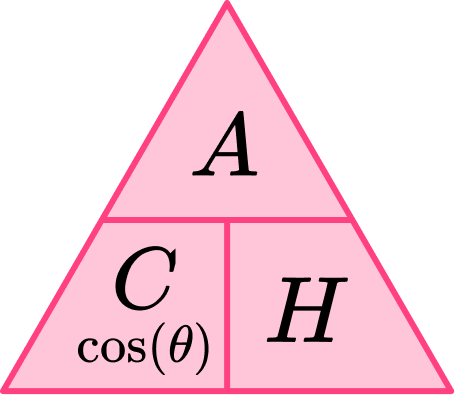\begin{aligned} x&=\frac{8}{\cos{51}}\\\\ &=12.71212583…\\\\ &=12.71 \ cm \ (2dp) \end{aligned}

2. Determine the size of the missing side marked \theta in the triangle below. Write your answer to 2 decimal places.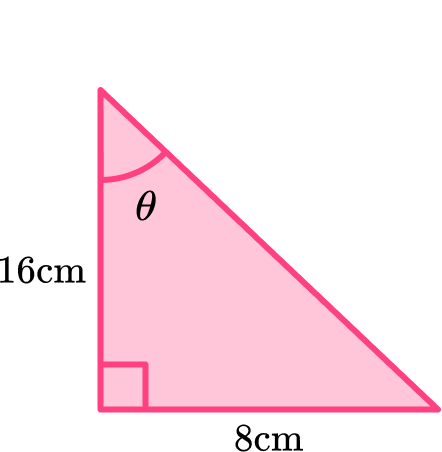\theta=26.57^{\circ}\theta=30.00^{\circ}\theta=60.00^{\circ}\theta=63.43^{\circ}After labelling the triangle with O, \ A and H,

• O=8 \ cm
• A=16 \ cm

SOH CAH TOA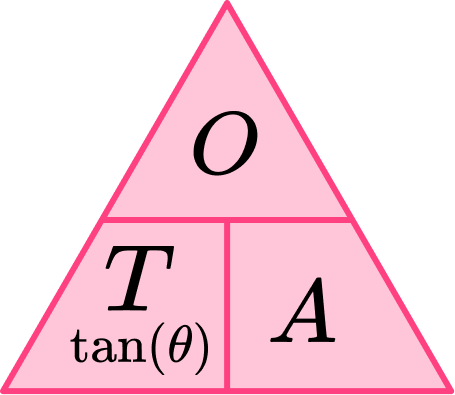\begin{aligned} \tan({\theta})&=\frac{8}{16}\\\\ \theta&=\tan^{-1}\left(\frac{8}{16}\right)\\\\ &=26.56505118…\\\\ &=26.57^{\circ} \ (2dp) \end{aligned}

3. Determine the size of the missing side marked \theta in the triangle below. Write your answer to a suitable degree of accuracy.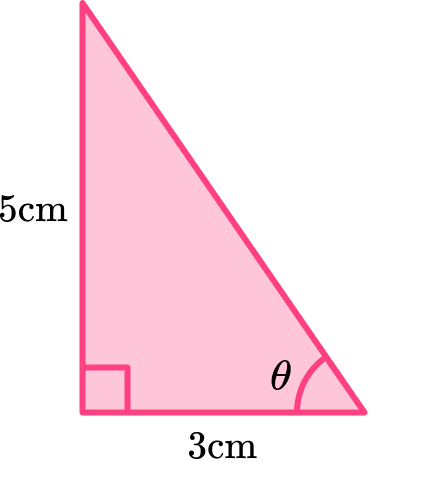\theta=0.01^{\circ}\theta=59.04^{\circ}\theta=0.03^{\circ}\theta=30.96^{\circ}After labelling the triangle with O, \ A and H,

• O=5 \ cm
• A=3 \ cm

SOH CAH TOA\begin{aligned} \tan({\theta})&=\frac{5}{3}\\\\ \theta&=\tan^{-1}\left(\frac{5}{3}\right)\\\\ &=59.03624347… \\\\ &=59.04^{\circ} \ (2dp) \end{aligned}

4. Determine the size of the missing length marked x in the triangle below. Write your answer to 2 decimal places.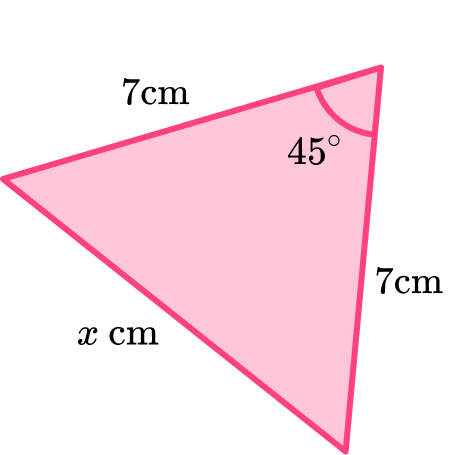x=7.00 \ cmx=4.95 \ cmx=5.36 \ cmx=28.70 \ cmLabelling the sides and angles, we have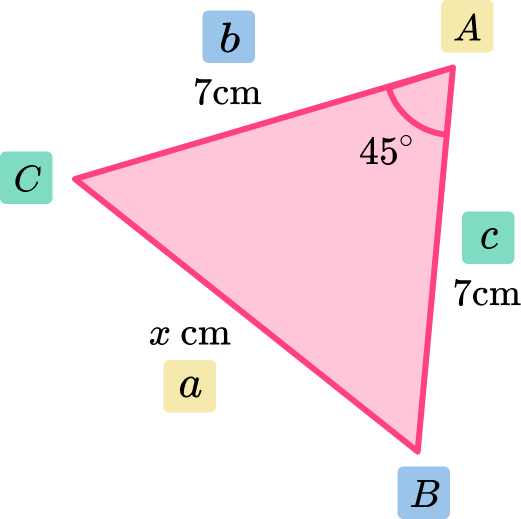As we know the two side lengths of b and c and the included angle at A, we need to use the cosine rule (the law of cosines) to determine the length of side x.

\begin{aligned} a^{2}&=b^{2}+c^{2}-2bc\cos(A)\\\\ x^{2}&=7^{2}+7^{2}-2\times{7}\times{7}\times\cos(45)\\\\ x^2&=98-98\cos(45)\\\\ x^2&=28.70353544…\\\\ x&=\sqrt{28.70353544…}\\\\ x&=5.357568053…\\\\ x&=5.36 \ cm \ (2dp) \end{aligned}

5. Determine the size of the missing angle marked \theta in the triangle below. Write your answer to 2 decimal places.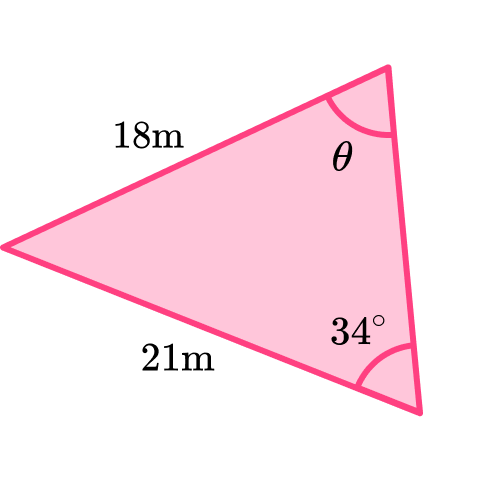\theta=40.72^{\circ}\theta=0.65^{\circ}\theta=11.76^{\circ}\theta=29.14^{\circ}Labelling the sides and angles, we have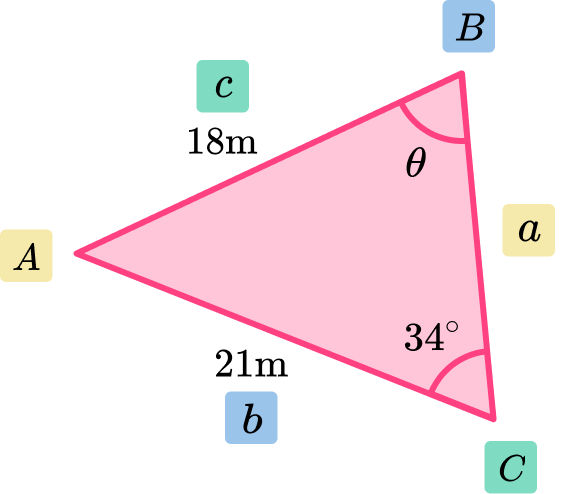As we know the angle at C the length of side c, the length of side b and we want to determine the angle at B, we need to use the sine rule (the law of sines) to determine the length of angle \theta.

Being careful with the labelling and the correct version of the sine rule, we have

\begin{aligned} \frac{\sin{B}}{b}&=\frac{\sin{C}}{c}\\\\ \frac{\sin{\theta}}{21}&=\frac{\sin{34}}{18}\\\\ \sin{\theta}&=\frac{\sin{34}}{18}\times{21}\\\\ \theta&=\sin^{-1}\left(\frac{21\sin{34}}{18}\right)\\\\ \theta&=40.72217103…\\\\ \theta&=40.72^{\circ} \ (2dp) \end{aligned}

6. Determine the area of the triangle below. Write your answer to 2 decimal places.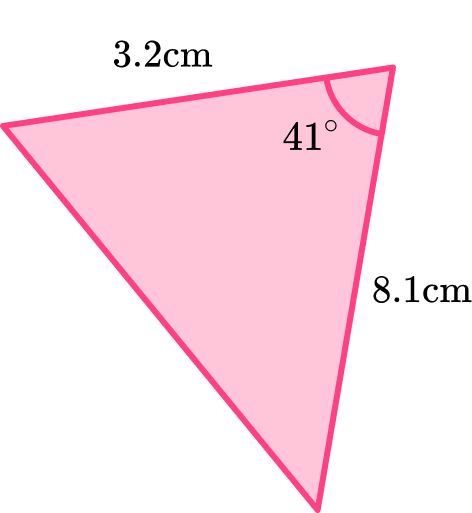6.06\text{ cm}^{2}12.96\text{ cm}^{2}68.44\text{ cm}^{2}8.50\text{ cm}^{2}As we know the included angle between two side lengths, we can find the area of a triangle using sine where

a=3.2 \ cm, \ b=8.1 \ cm and C=41^{\circ}.

Being careful with the labelling and the correct version of the sine rule, we have

\begin{aligned} A&=\frac{1}{2}ab\sin{C}\\\\ &=\frac{1}{2}\times{3.2}\times{8.1}\times\sin{41}\\\\ &=12.96\sin{41}\\\\ &=8.502525016… \\\\ A&=8.50 \ cm^2 \ (2dp) \end{aligned}

### Trigonometry formulas GCSE questions

1. Using the triangle below, complete the table. Give your answers in exact form.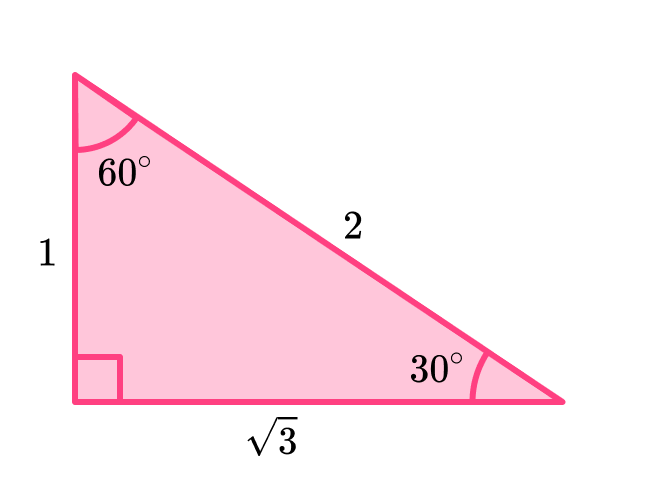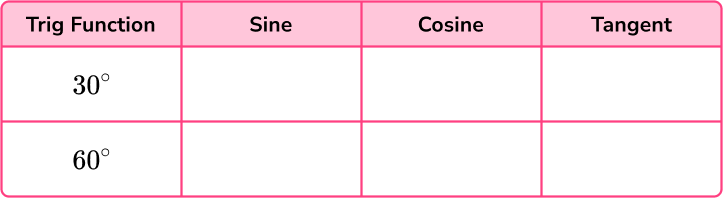(3 marks)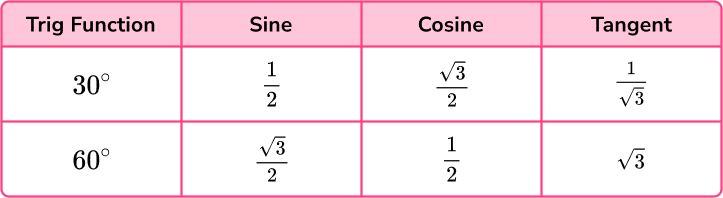6 correct

(1)

5 correct

(1)

4 correct

(1)

2. (a) Rearrange the equation \theta=\sin^{-1}(\frac{O}{H}) to make H the subject.

(b) Rearrange the equation A=\frac{1}{2}ab\sin{C} to make b the subject.

(c) Using your answer to part b) or otherwise, determine the missing length of the triangle ABC below. Write your answer to 2 decimal places.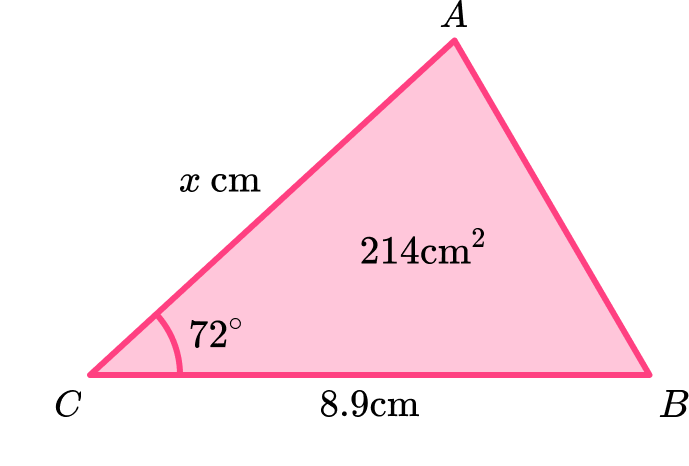(7 marks)

(a)

\sin(\theta)=\frac{O}{H}

(1)

H\sin(\theta)=O

(1)

H=\frac{O}{\sin(\theta)}

(1)

(b)

2A=ab\sin{C}

(1)

\frac{2A}{a\sin{C}}=b

(1)

(c)

b=\frac{2\times214}{8.9\sin72}

(1)

b=50.56 \ cm \ (2dp)

(1)

3. (a) Show that the formula \frac{a}{\sin{A}}=\frac{b}{\sin{B}} rearranges to make B=\sin^{-1}(\frac{b\sin{A}}{a}).

(b) Determine the size of the missing angle \theta in the triangle below. Write your answer to 2 decimal places.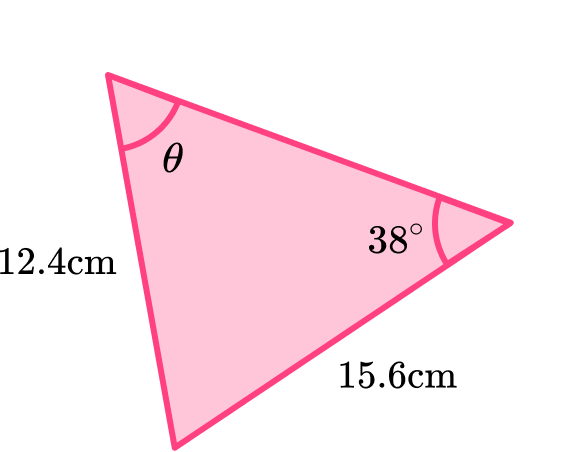(5 marks)

(a)

a\sin{B}=b\sin{A}

(1)

\sin{B}=\frac{b\sin{A}}{a}

(1)

B=\sin^{-1}(\frac{b\sin{A}}{a})

(1)

(b)

\theta =\sin^{-1}(\frac{b\sin{A}}{a})=\sin^{-1}(\frac{15.6\sin38}{12.4})

(1)

\theta=50.76^{\circ}

(1)

4. (a) Show that the formula a^2=b^2+c^2-2bc\cos{A} rearranges to make A=\cos^{-1}(\frac{b^2+c^2-a^2}{2bc}).

(b) Determine the size of the missing angle \theta in the triangle below. Write your answer to 2 decimal places.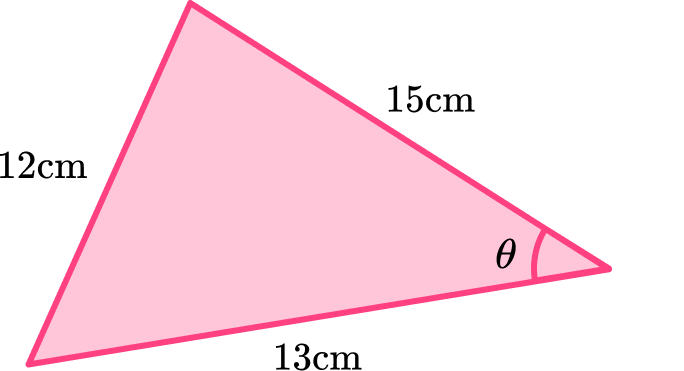(5 marks)

(a)

2bc\cos{A}=b^2+c^2-a^2

(1)

\cos{A}=\frac{b^2+c^2-a^2}{2bc}

(1)

A=\cos^{-1}(\frac{b^2+c^2-a^2}{2bc})

(1)

(b)

\theta =\cos^{-1}(\frac{b^2+c^2-a^2}{2bc})=\cos^{-1}(\frac{13^2+15^2-12^2}{2\times13\times15})

(1)

\theta=50.13^{\circ}

(1)

## Learning checklist

You have now learned how to:

• Extend and formalise knowledge of ratio and proportion, including trigonometric ratios, in working with measures and geometry, and in working with proportional relations algebraically
• Know and apply the sine rule, \frac{a}{\sin{A}}=\frac{b}{\sin{B}}=\frac{c}{\sin{C}}, and cosine rule, a^2=b^2+c^2-2bc\cos{A}, to find unknown lengths and angles
• Know and apply Area = \frac{1}{2}ab\sin{C} to calculate the area, sides or angles of any triangle

## Beyond GCSE

Trigonometry is a large branch of mathematics. At GCSE, you barely scratch the surface of how widely trigonometry is used within mathematics and so this is developed further at A level. Other trigonometric functions you will look at at A level include

• Secant, cosecant and cotangent reciprocal identities
• Secant or sec \theta \ (\sec\theta=\frac{1}{\sin{\theta}})
• Cosecant or cosec \theta \ (\csc\theta=\frac{1}{\cos{\theta}})
• Cotangent or cot \theta \ (\cot{\theta}=\frac{1}{\tan{\theta}})

• Double angle formulas (the angle sum and difference formulas/sum and difference identities)
• \sin(A\pm{B})=\sin{A}\cos{B}\pm\cos{A}\sin{B}
• \cos(A\pm{B})=\cos{A}\cos{B}\mp\sin{A}\sin{B}
• \tan(A\pm{B})=\frac{\tan{A}\pm\tan{B}}{1\mp\tan{A}\tan{B}}

• Half angle identities
• \sin\left(\frac{A}{2}\right)=\pm\sqrt{\frac{1-\cos{A}}{2}}
• \cos\left(\frac{A}{2}\right)=\pm\sqrt{\frac{1+\cos{A}}{2}}
• \tan\left(\frac{A}{2}\right)=\pm\sqrt{\frac{1-\cos{A}}{1+\cos{A}}}

• Product identities
• \cos{A}\cos{B}=\frac{1}{2}[\cos(A+B)+\cos(A-B)]
• \sin{A}\sin{B}=\frac{1}{2}[\cos(A-B)-\cos(A+B)]
• \sin{A}\cos{B}=\frac{1}{2}[\sin(A+B)+\sin(A-B)]
• \cos{A}\sin{B}=\frac{1}{2}[\sin(A+B)-\sin(A-B)]

• Pythagorean identities
• \sin^{2}\theta+\cos^{2}\theta=1
• \sin^{2}\theta=1-\cos^{2}\theta
• \sin\theta=\sqrt{1-\cos^{2}\theta}

We also consider the graphs of the functions of \sin \ \theta, \ \cos \ \theta, and \tan \ \theta in greater detail in relation to when the functions are positive and negative for angles and the periodicity beyond the range of 0^{\circ} \leq \theta \leq 180^{\circ} by using the four quadrants of an xy set of axes as well as using a different unit of measure for angles, radians. This also includes the periodicity identities for trigonometric functions in radians.

## Still stuck?

Prepare your KS4 students for maths GCSEs success with Third Space Learning. Weekly online one to one GCSE maths revision lessons delivered by expert maths tutors.

Find out more about our GCSE maths tuition programme.